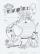Team

The football team has 11 players. Their average age is 20 years. During the match due to injury, one player withdraws and the average age of the team is 18.

How old was injured player?

Result

x =  40

Solution:(s+x)/11=20
s/10=18

s+x = 220
s = 180

s = 180
x = 40

Calculated by our linear equations calculator.

Leave us a comment of example and its solution (i.e. if it is still somewhat unclear...):Be the first to comment!To solve this example are needed these knowledge from mathematics:

Looking for help with calculating arithmetic mean? Looking for a statistical calculator? Do you have a linear equation or system of equations and looking for its solution? Or do you have quadratic equation?

Next similar examples:

1. Two numbersWe have two numbers. Their sum is 140. One-fifth of the first number is equal to half the second number. Determine those unknown numbers.
2. Exam averageAverage of marks that have on the certificates students of 8.A class in mathematics is exactly 2.45. If we did not add 1 and 3 of sutdents Michael and Alena, who arrived a month ago, it would average exactly 2.5. Determine how many students have class 8.
3. Ball gameRichard, Denis and Denise together scored 932 goals. Denis scored 4 goals over Denise but Denis scored 24 goals less than Richard. Determine the number of goals for each player.
4. Three brothersThe three brothers have a total of 42 years. Jan is five years younger than Peter and Peter is 2 years younger than Michael. How many years has each of them?
5. Linsys2Solve two equations with two unknowns: 400x+120y=147.2 350x+200y=144
6. BlackberriesDaniel, Jolana and Stano collected together 34 blackberries. Daniel collected 8 blackberries more than Jolana, Jolana 4 more than Stano. Determine the number blackberries each collected .
7. TheatroTheatrical performance was attended by 480 spectators. Women were in the audience 40 more than men and children 60 less than half of adult spectators. How many men, women and children attended a theater performance?
8. Vijuviju has 40 chickens and rabbits. If in all there are 90 legs. How many rabbits are there with viju??
9. Walnutsx walnuts were in the mission. Dano took 1/4 of nuts Michael took 1/8 from the rest and John took 34 nuts. It stayed here 29 nuts. Determine the original number of nuts.
10. Theorem proveWe want to prove the sentence: If the natural number n is divisible by six, then n is divisible by three. From what assumption we started?
11. Factory and divisionsThe factory consists of three auxiliary divisions total 2,406 employees. The second division has 76 employees less than 1st division and 3rd division has 212 employees more than the 2nd. How many employees has each division?
12. Fifth of the numberThe fifth of the number is by 24 less than that number. What is the number?
13. TreesAlong the road were planted 250 trees of two types. Cherry for 60 CZK apiece and apple 50 CZK apiece. The entire plantation cost 12,800 CZK. How many was cherries and apples?
14. Elimination methodSolve system of linear equations by elimination method: 5/2x + 3/5y= 4/15 1/2x + 2/5y= 2/15
15. Substitutionsolve equations by substitution: x+y= 11 y=5x-25
16. SchoolsThree schools are attended by 678 pupils. To the first attend 21 students more and to the third 108 fewer students than to second school. How many students attend the schools?
17. Three figures - numbersThe sum of three numbers, if each is 10% larger than the previous one, is 662. Determine the figures.